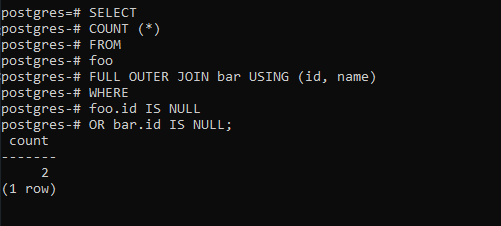# PostgreSQL – Comparing Tables

In PostgreSQL, there are several ways to compare two tables to find the difference between them. In this article, we will look into the two most commonly used techniques.

1. Comparison using EXCEPT and UNION operators

First, let’s create table two tables named foo and bar, and insert some sample data for the demonstration.

```CREATE TABLE foo (
ID INT PRIMARY KEY,
NAME VARCHAR (50)
);
INSERT INTO foo (ID, NAME)
VALUES
(1, 'a'),
(2, 'b');

```
```CREATE TABLE bar (
ID INT PRIMARY KEY,
NAME VARCHAR (50)
);
INSERT INTO bar (ID, NAME)
VALUES
(1, 'a'),
(2, 'b');

```

The foo table has the same structure and data as the bar table.

Next, we update one row in the bar table.

```UPDATE bar
SET name = 'c'
WHERE
id = 2;

```

Now our tables look like below: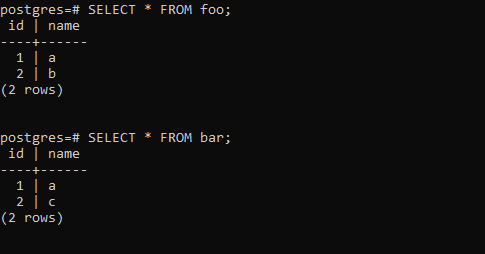Then, to find the rows in the foo table but not in the bar table, we use the following query:

```SELECT
ID,
NAME,
'not in bar' AS note
FROM
foo
EXCEPT
SELECT
ID,
NAME,
'not in bar' AS note
FROM
bar;

```

Output: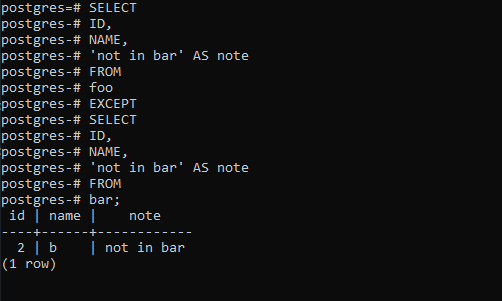We used EXCEPT operator that returns the rows in the foo table but not in the bar table. We can apply the same technique to find the rows that are in the bar table but not in the foo table.

```SELECT
ID,
NAME,
'not in foo' AS note
FROM
bar
EXCEPT
SELECT
ID,
NAME,
'not in foo' AS note
FROM
foo;

```

Output: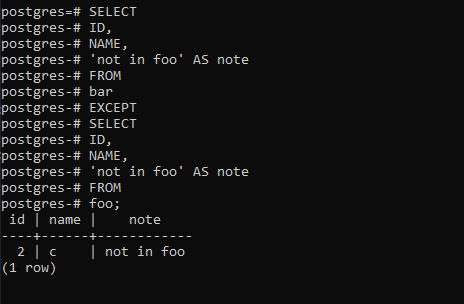2. Comparison using OUTER JOIN

OUTER JOIN can be used to compare two tables as follows:

```SELECT
id,
name
FROM
foo
FULL OUTER JOIN bar USING (id, name)
WHERE
foo.id IS NULL
OR bar.id IS NULL;
```

Output: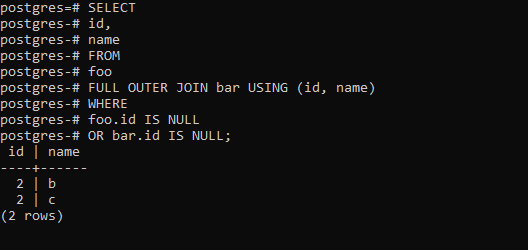To find the number of rows that are in the foo table but not bar table and vice versa, we use the COUNT function as follows:

```SELECT
COUNT (*)
FROM
foo
FULL OUTER JOIN bar USING (id, name)
WHERE
foo.id IS NULL
OR bar.id IS NULL;
```

Output: